Exam 1998b pdfLoading previews...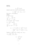integration by parts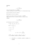integration using the trapezium rule and Simpson's rule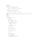first order differential equations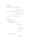second order linear differential equations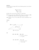vector equations of lines and planes
7 files in this resource

Exam 1998b pdf

Exam and solutions in PDFView Item